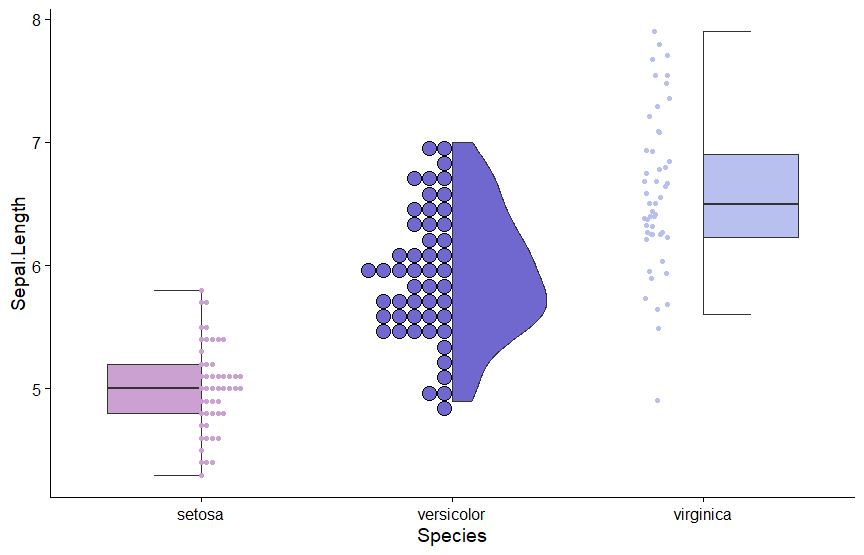# Using gghalves

This vignette is intended to showcase the usage of the gghalves extension by going through the individual _half_ geoms to explain details of usage and function arguments.

## General Idea

The general idea of gghalves stems from this StackOverflow question on how to plot a hybrid boxplot. This led to me developing the ggpol extension for ggplot2. However, the fact that ggpol has become a sort of aggregation for all kinds of geoms over time, and seeing that many things can be cut in half, has ultimately led to this library.

The idea is that many geoms that aggregate data, such as geom_boxplot, geom_violin and geom_dotplot are (near) symmetric. Given that the space to display information is limited, we can make better use of it by cutting the geoms in half and displaying additional geoms that e.g. give information about the sample size.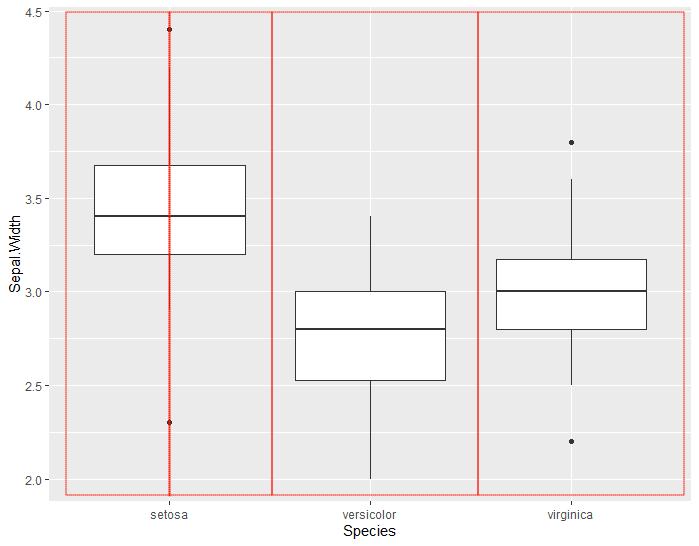## GeomHalfPoint

GeomHalfPoint, perhaps counterintuitively, does not display a literal half-circle. Rather, it plots the data points such that

• the space they occupy is at most half of the space allotted to the specific factor, on the x-axis
• they leave the left or right half of the total space to be used by another _half_ geom

Further, by default geom_half_point jitters the points horizontally and vertically.

ggplot(iris, aes(x = Species, y = Sepal.Width)) +
geom_half_point()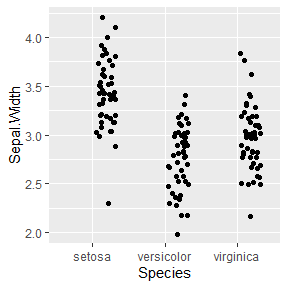The way this works is that transformation = PositionJitter is passed to the geom. We could play with the default values of this transformation by passing along a transformation_params argument

ggplot(iris, aes(x = Species, y = Sepal.Width)) +
geom_half_point(transformation_params = list(height = 0, width = 0.001, seed = 1))
#> Warning in geom_half_point(transformation_params = list(height = 0, width =
#> 0.001, : Ignoring unknown parameters: transformation_params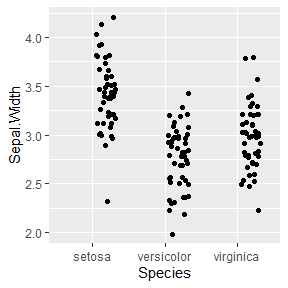or we could change the transformation argument itself:

ggplot(iris, aes(x = Species, y = Sepal.Width)) +
geom_half_point(transformation = PositionIdentity)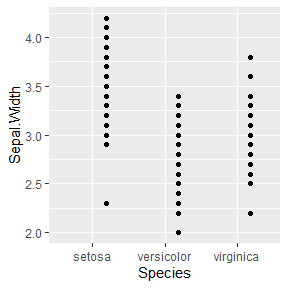Making the transformation work with custom Positions from ggplot2 extensions is something that will hopefully be included in future updates of this package.

## GeomHalfPointPanel

Sometimes we want to color points within the aes() groupings. In that case, we can make use of geom_half_point_panel().

ggplot(iris, aes(y = Sepal.Width)) +
geom_half_boxplot() +
geom_half_point_panel(aes(x = 0.5, color = Species), range_scale = .5)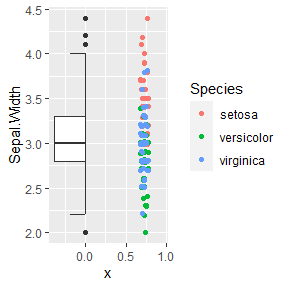Like all _half_ geoms, geom_half_point also takes a side argument, with l for left and r for right.

## GeomHalfBoxplot

GeomHalfBoxplot displays a boxplot that is cut in half and plotted either on the left or right side of the space allotted to the specific factor on the x-axis.

ggplot(iris, aes(x = Species, y = Sepal.Width)) +
geom_half_boxplot()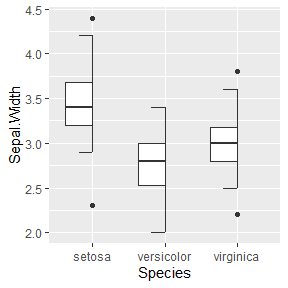Additionally to the standard side argument, you can also center the half-boxplot and decide whether an errorbar is drawn or not.

ggplot(iris, aes(x = Species, y = Sepal.Width)) +
geom_half_boxplot(side = "r", center = TRUE, errorbar.draw = FALSE)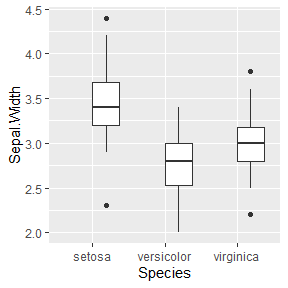## GeomHalfViolin

GeomHalfViolin draws a half-violin plot. Besides the side argument, it supports all the arguments that can be passed to the standard GeomViolin.

ggplot(iris, aes(x = Species, y = Sepal.Width)) +
geom_half_violin()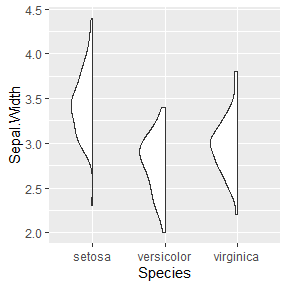Furthermore, if we have a binary grouping variable (such as control/treatment) we can plot side-by-side comparisons with the optional split aesthetic:

ggplot() +
geom_half_violin(
data = ToothGrowth,
aes(x = as.factor(dose), y = len, split = supp, fill = supp),
position = "identity"
)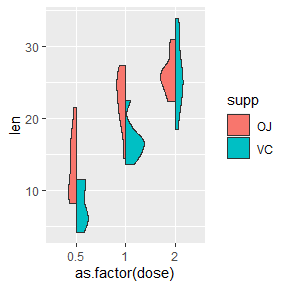## GeomHalfDotplot

GeomHalfDotplot is slightly different from the other _half_ geoms in that it does not support a side argument, since this is already inherently built into the standard GeomDotplot via stackdir:

ggplot(iris, aes(x = Species, y = Sepal.Width)) +
geom_half_violin() +
geom_dotplot(binaxis = "y", method="histodot", stackdir="up")
#> Bin width defaults to 1/30 of the range of the data. Pick better value with
#> binwidth.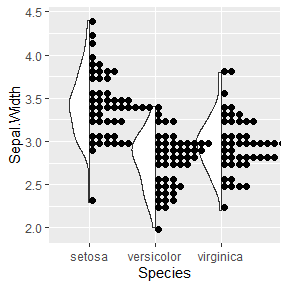So, given that geom_dotplot can be used as a _half_ geom, why the need for geom_half_dotplot? The reason is that geom_dotplot does not support dodging when there are multiple factors in play. Let’s consider the following example:

df <- data.frame(score = rgamma(150, 4, 1),
gender = sample(c("M", "F"), 150, replace = TRUE),
genotype = factor(sample(1:3, 150, replace = TRUE)))

Given this data, we want to group by genotype, but also separate the plots by gender. This does not quite work using the standard geom:

ggplot(df, aes(x = genotype, y = score, fill = gender)) +
geom_half_violin() +
geom_dotplot(binaxis = "y", method="histodot", stackdir="up", position = PositionDodge)
#> Bin width defaults to 1/30 of the range of the data. Pick better value with
#> binwidth.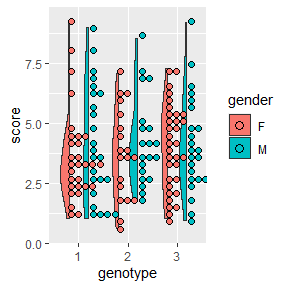Using geom_half_dotplot, however, we can make this work:

ggplot(df, aes(x = genotype, y = score, fill = gender)) +
geom_half_violin() +
geom_half_dotplot(method="histodot", stackdir="up")
#> Bin width defaults to 1/30 of the range of the data. Pick better value with
#> binwidth.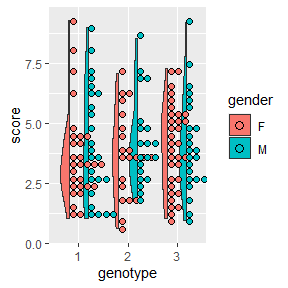## Working with ggplot2 Extensions

As mentioned in the package description, gghalves can work well in combination with certain ggplot2 extensions. One of them is geom_beeswarm of the ggbeeswarm package. Note that, currently, you will need to install the latest version from GitHub to support the passing of beeswarmArgs.

ggplot(iris, aes(x = Species, y = Sepal.Width)) +
geom_half_boxplot() +
geom_beeswarm(beeswarmArgs = list(side = 1))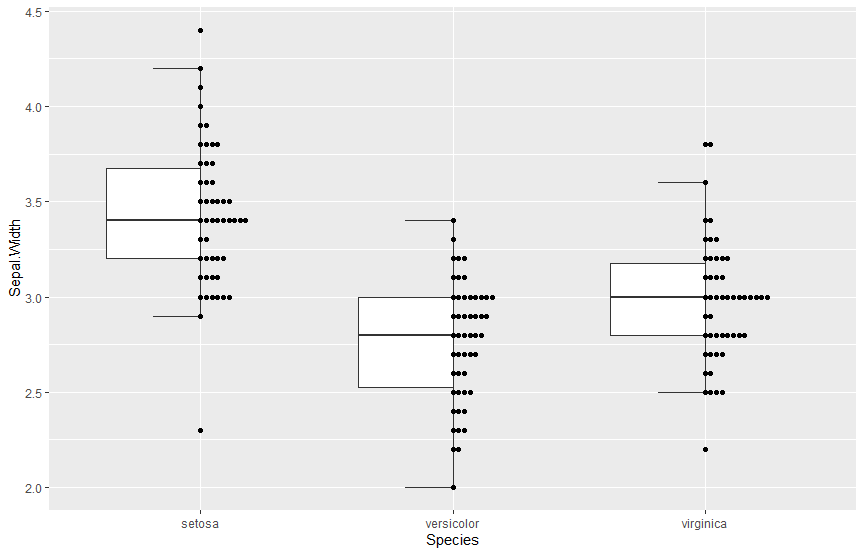## Combining Different Geoms

Lastly, let us remake the plot displayed in the GitHub Readme. It is for display-purposes only, and thus uses a lot of filtering and a lot of geoms…

ggplot() +

geom_half_boxplot(
data = iris %>% filter(Species=="setosa"),
aes(x = Species, y = Sepal.Length, fill = Species), outlier.color = NA) +

ggbeeswarm::geom_beeswarm(
data = iris %>% filter(Species=="setosa"),
aes(x = Species, y = Sepal.Length, fill = Species, color = Species), beeswarmArgs=list(side=+1)
) +

geom_half_violin(
data = iris %>% filter(Species=="versicolor"),
aes(x = Species, y = Sepal.Length, fill = Species), side="r") +

geom_half_dotplot(
data = iris %>% filter(Species=="versicolor"),
aes(x = Species, y = Sepal.Length, fill = Species), method="histodot", stackdir="down") +

geom_half_boxplot(
data = iris %>% filter(Species=="virginica"),
aes(x = Species, y = Sepal.Length, fill = Species), side = "r", errorbar.draw = TRUE,
outlier.color = NA) +

geom_half_point(
data = iris %>% filter(Species=="virginica"),
aes(x = Species, y = Sepal.Length, fill = Species, color = Species), side = "l") +

scale_fill_manual(values = c("setosa" = "#cba1d2", "versicolor"="#7067CF","virginica"="#B7C0EE")) +
scale_color_manual(values = c("setosa" = "#cba1d2", "versicolor"="#7067CF","virginica"="#B7C0EE")) +
theme(legend.position = "none")